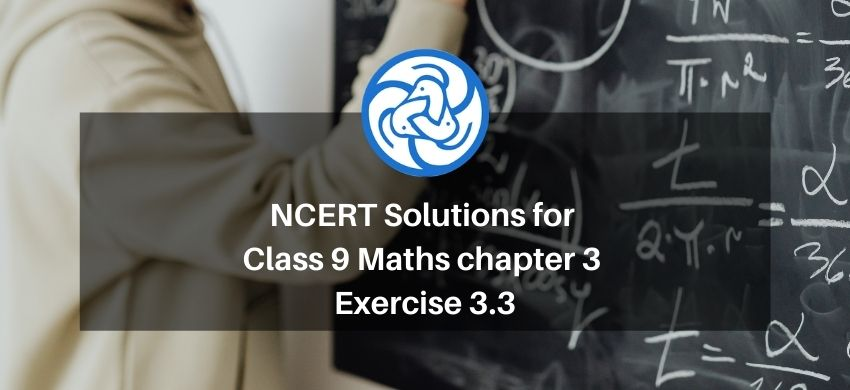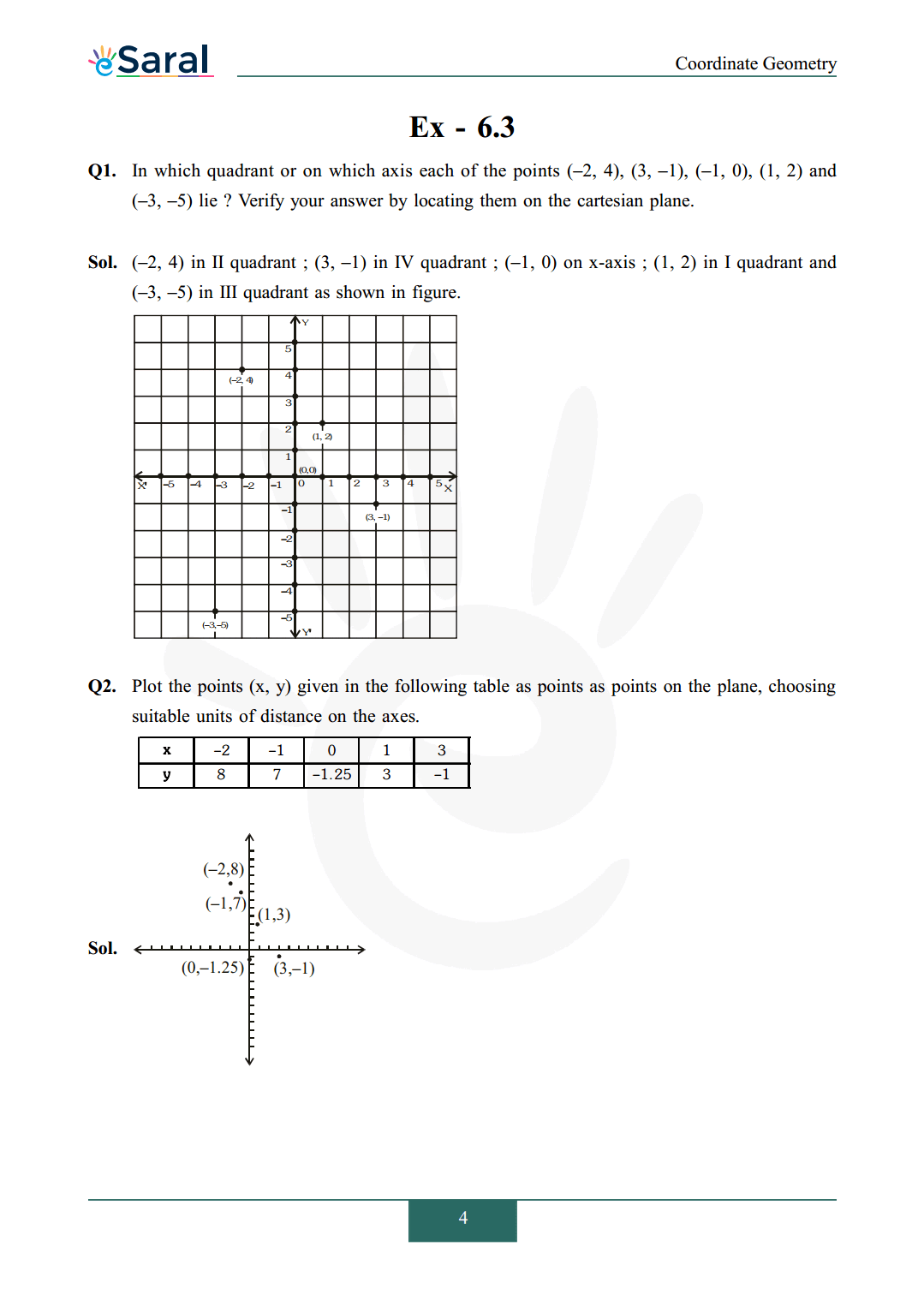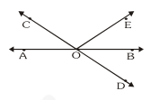Most Affordable JEE | NEET | 8,9,10 Preparation by Kota's Top IITian Doctor Faculties

# NCERT Solutions for Class 9 Maths chapter 3 Exercise 3.3 - Coordinate Geometry - Free PDF DownloadHey, are you a class 9 Student and Looking for Ways to Download NCERT Solutions for Class 9 Maths chapter 3 Exercise 3.3? If Yes then you are at the right place.

Here we have listed Class 9 maths chapter 3 exercise 3.3 solutions in PDF that is prepared by Kota’s top IITian’s Faculties by keeping Simplicity in mind.

If you want to score high in your class 9 Maths Exam then it is very important for you to have a good knowledge of all the important topics, so to learn and practice those topics you can use eSaral NCERT Solutions.

So, without wasting more time Let’s start.

### Download The PDF of NCERT Solutions for Class 9 Maths chapter 3 Exercise 3.3 "Coordinate Geometry"#### All Questions of Chapter 3 Exercise 3.3

Once you complete the chapter 3 then you can revise Ex. 3.3 by solving following questions

Q1. In which quadrant or on which axis each of the points $(-2,4),(3,-1),(-1,0),(1,2)$ and $(-3,-5)$ lie ? Verify your answer by locating them on the cartesian plane.

Q2. Plot the points (x, y) given in the following table as points as points on the plane, choosing suitable units of distance on the axes.Also Read,